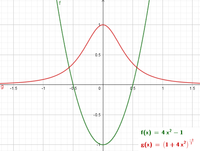# Area between two curves integral

#### dBanji

##### New member
Hi everyone, please I need help with this problem. I am stuck. See below
Can someone please help with this problem: Approximate the points at which the graphs of f(x) = 4x^2 − 1 and g(x) = (1 + 4x^2)^(−3/2) intersect, and approximate the area (in units^2) between their graphs accurate to three decimal places.

I used a graphing calculator to plot the graph and found the point of intersections to be approx x=0.6 and -0.6. Then I found the antiderivative of f(x) as (4x^3)/3 -x. the antiderivative of g(x) I found to be [-(1+4x^2)^(-1/2)]/4x. I did not include +C because it's definite integral from -0.6 to 0.6. I subtracted the lower function f(x) from the upper function g(x) i.e. g(x) - f(x) and got 0.065 but that was wrong. What else should I do? I need someone to help, please.

Thanks.

Last edited:

#### ISTER_REG

##### New member
The approx intersections are at $$\displaystyle x \approx \pm 0.56763$$. Next step is $$\displaystyle \int_a^b (\textcolor{red}{top} - \textcolor{green}{bottom} ) dx = area$$. In your case the top function is $$\displaystyle \textcolor{red}{g(x) = (1+4x^2)^{-\frac{3}{2}}}$$ and the bottom function is $$\displaystyle \textcolor{green}{f(x) = 4x^2-1}$$. The integral is as follows $$\displaystyle \int_{-0.56763}^{0.56763} \left(g(x) -f(x)\right) \, dx = \int_{-0.56763}^{0.56763} \left((1+4x^2)^{-\frac{3}{2}} -(4x^2-1)\right) \, dx \approx 1.39794$$Short side question: Is there additionally asked for a method to determine the intersection point? I mean you can do that graphically, via calculator, but analytically or approximatively goes also...

Last edited:
•dBanji and nasi112

#### dBanji

##### New member
The approx intersections are at $$\displaystyle x \approx \pm 0.56763$$. Next step is $$\displaystyle \int_a^b (\textcolor{red}{top} - \textcolor{green}{bottom} ) dx = area$$. In your case the top function is $$\displaystyle \textcolor{red}{g(x) = (1+4x^2)^{-\frac{3}{2}}}$$ and the bottom function is $$\displaystyle \textcolor{green}{f(x) = 4x^2-1}$$. The integral is as follows $$\displaystyle \int_{-0.56763}^{0.56763} \left(g(x) -f(x)\right) \, dx = \int_{-0.56763}^{0.56763} \left((1+4x^2)^{-\frac{3}{2}} -(4x^2-1)\right) \, dx \approx 1.39794$$

View attachment 26621

Short side question: Is there additionally asked for a method to determine the intersection point? I mean you can do that graphically, via calculator, but analytically or approximatively goes also...
I cannot thank you enough. I am so grateful. The answer is correct now. I did not use the calculator to integrate rather I worked it out. I had the correct result for the f(x) but the g(x) was wrong. I think the problem was the integration process. How do you integrate g(x) without using a calculator.
Thank you for finding time to help. I appreciate it.

And what software did you use to type those integrations and graph? I'd like to have it as well for clarity of explanation or question.

#### ISTER_REG

##### New member
No problemFor the plot I used a software called Desmos, here is a link for the online plotter: https://www.desmos.com/calculator?lang=en

It is possible to integrate this via hand, but therefore one have to use for example the (not obvious) substitution $$\displaystyle x = \frac{\tan(u)}{2}$$

#### dBanji

##### New member
thank you very much